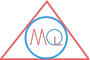# List of Symbols Used in Geometry

## Symbols used in Geometry

$$^o$$Degree$$45^o$$
$$\angle$$Angle$$\angle 45^o$$
$$\bot$$Perpendicular$$AB \bot CD$$
$$||$$Parallel$$AB || CD$$
$$\triangle$$Triangle$$\triangle ABC$$
$$\sim$$Similar to$$\triangle ABC \sim \triangle PQR$$
$$\cong$$Congurent to$$\triangle ABC \cong \triangle PQR$$